Get inspired by the success stories of our students in IIT JAM MS, ISI  MStat, CMI MSc DS.  Learn More

# Collect All The Toys | ISI MStat 2013 PSB Problem 9Remember, we used to collect all the toy species from our chips' packets. We were all confused about how many more chips to buy? Here is how, probability guides us through in this ISI MStat 2013 Problem 9.

## Problem

Chips are on sale for Rs. 30 each. Each chip contains exactly one toy, which can be one of four types with equal probability. Suppose you keep on buying chips and stop when you collect all the four types of toys. What will be your expected expenditure?

## Solution

I am really excited to find the solution. Remember, in your childhood, you were excited to buy packets of chips to collect all the toys and show them to your friends. There was a problem, that you don't have enough money to buy the chips. A natural question was how much money you have to spend?

See, the problem is right here! Let's dive into the solution. Remember we have to be a little bit mathematical here.

Let's see how we get the four new toys.

1st new toy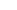2nd new toy3rd new toy4th new toy.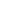= The number of chips to be bought to get the first new toy.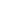= The number of chips to be bought to get the second new toy after you got the first new toy.= The number of chips to be bought to get the third new toy after you got the second new toy.= The number of chips to be bought to get the fourth new toy after you got the third new toy.

Observe that the expected number of chips to be bought =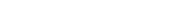=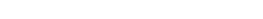.

Now, can you guess what are the random variables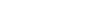?

There are all geometric random variables. ( Why? )

[ Hint : Success is when you don't get the already observed toys ]

Observe that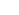~ Geom(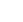) ( Why? )

If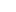~ Geom(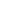), then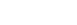.

Therefore, the required number of chips to be brought is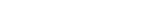.

Therefore, you can guess, what will be the answer if there are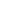toys? (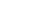) , where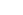is the nth harmonic number.

## Simulation and Verification

v = NULL
N = 0
n = 4  #number of toys
init = rep(0,n)
toy = rep(0,n)
True = rep(TRUE,n)
for (j in 1:10000)
{
for (i in 1:100)
{
s = sample(4,1)
toy[s] = toy[s] + 1
N = N + 1
if (identical (toy > init, True))
{
break
}
}
v = c(v,N)
N = 0
toy = rep(0,n)
}
mean(v) = # 8.3214


Therefore, it is verified by simulation.This is the histogram of the mean, which is expected to be around 8.33

Stay Tuned! Stay Shambho!

Remember, we used to collect all the toy species from our chips' packets. We were all confused about how many more chips to buy? Here is how, probability guides us through in this ISI MStat 2013 Problem 9.

## Problem

Chips are on sale for Rs. 30 each. Each chip contains exactly one toy, which can be one of four types with equal probability. Suppose you keep on buying chips and stop when you collect all the four types of toys. What will be your expected expenditure?

## Solution

I am really excited to find the solution. Remember, in your childhood, you were excited to buy packets of chips to collect all the toys and show them to your friends. There was a problem, that you don't have enough money to buy the chips. A natural question was how much money you have to spend?

See, the problem is right here! Let's dive into the solution. Remember we have to be a little bit mathematical here.

Let's see how we get the four new toys.

1st new toy2nd new toy3rd new toy4th new toy.= The number of chips to be bought to get the first new toy.= The number of chips to be bought to get the second new toy after you got the first new toy.= The number of chips to be bought to get the third new toy after you got the second new toy.= The number of chips to be bought to get the fourth new toy after you got the third new toy.

Observe that the expected number of chips to be bought ==.

Now, can you guess what are the random variables?

There are all geometric random variables. ( Why? )

[ Hint : Success is when you don't get the already observed toys ]

Observe that~ Geom() ( Why? )

If~ Geom(), then.

Therefore, the required number of chips to be brought is.

Therefore, you can guess, what will be the answer if there aretoys? () , whereis the nth harmonic number.

## Simulation and Verification

v = NULL
N = 0
n = 4  #number of toys
init = rep(0,n)
toy = rep(0,n)
True = rep(TRUE,n)
for (j in 1:10000)
{
for (i in 1:100)
{
s = sample(4,1)
toy[s] = toy[s] + 1
N = N + 1
if (identical (toy > init, True))
{
break
}
}
v = c(v,N)
N = 0
toy = rep(0,n)
}
mean(v) = # 8.3214


Therefore, it is verified by simulation.This is the histogram of the mean, which is expected to be around 8.33

Stay Tuned! Stay Shambho!

This site uses Akismet to reduce spam. Learn how your comment data is processed.

### Knowledge Partner